Courses

# Past Year Questions: Friction Notes | EduRev

## Mechanical Engineering : Past Year Questions: Friction Notes | EduRev

The document Past Year Questions: Friction Notes | EduRev is a part of the Mechanical Engineering Course Engineering Mechanics.
All you need of Mechanical Engineering at this link: Mechanical Engineering

Question 1:A wardrobe(mass 100 kg, height4m, width 2 m, depth 1 m), symmetric about the Y-Y axis, stands on a rough level floor as shown in the figure. A force P is applied at mid-height on the wardrobe so as to tip it about point Q without slipping. What are the minimum values of the force (in newton) and the static coefficient of friction μ between the floor and the wardrobe, respectively?

[2014, Set-4]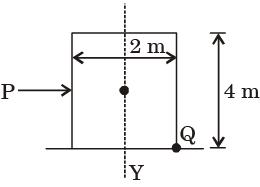Question 2:A block R of mass 100 kg is placed on a block S of mass 150 kg as shown in the figure. Block R is tied to the wall by a massless and inextensible string PQ. If the coefficient of static friction for all surfaces is 0.4, the minimum force F(in kN) needed to move the block S is

[2014, Set-1]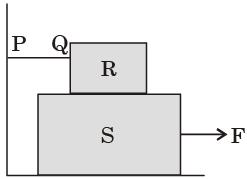Question 3:​A 1 kg block is resting on a surface with coefficient of friction m = 0.1. A force of 0.8 N is applied to the block as shown in the figure. The friction force is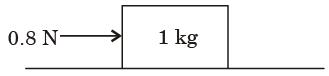Question 4:​A block weighing 981 N is resting on a horizontal surface. The coefficient of friction between the block and the horizontal surface is m = 0.2. A vertical cable attached to the block provides partial support as shown. A man can pull horizontally with a force of 100 N. What will be the tension, T (in N) in the cable if the man is just able to move the block to the right?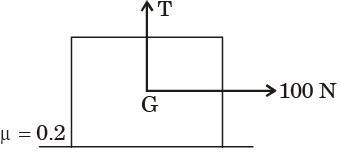Question 5:A block of mass M is released from point P on a rough inclined plane with inclination angle θ, shown in the figure below. The coefficient of friction is μ. If μ < tan θ, then the time taken by the block to reach another point Q on the inclined plane, where PQ = s, is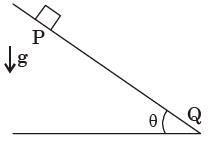Question 6:Two books of mass 1 kg each are kept on a table, one over the other. The coefficient of friction on every pair of contacting surfaces is 0.3. The lower book is pulled with a horizontal force F. The minimum value of F for which slip occurs between the two books is



Question 7:The figure shows a pair of pin-jointed grippertongs holding an object weighing 2000 N. The coefficient of friction (μ) at the gripping surface is 0.1. XX is the line of action of the input force and YY is the line of application of gripping force. If the pin- joint is assumed to be frictionless, the magnitude of force F required to hold the weight is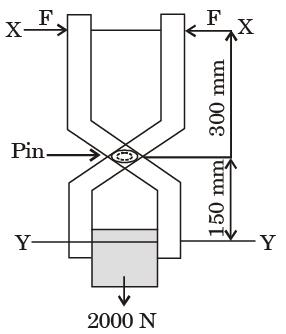Question 8:The ratio of tension on the tight side to that of the slack ride in a flat belt drive is



Question 9:A steel wheel of 600 mm diameter on a horizontal steel rail. It carries a load of 500 N. The coefficient of rolling resistance is 0.3 The force in Newton, necessary to roll the wheel along the rail is



Question 10:A wheel of mass m and radius r is in accelerated rolling motion without slip under a steady axle torque T. If the coefficient of kinetic friction is μ, the friction force from the ground on the wheel is



Offer running on EduRev: Apply code STAYHOME200 to get INR 200 off on our premium plan EduRev Infinity!

## Engineering Mechanics

37 videos|85 docs|64 tests

,

,

,

,

,

,

,

,

,

,

,

,

,

,

,

,

,

,

,

,

,

;